# ACCESSORIES

52 products found
Filtres

Gender

Size

Price

€0.00 - €40.00

Couleurs

Sport

Use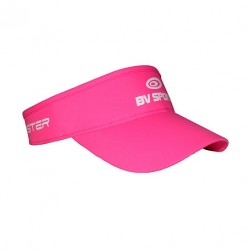-€10.00
• One Size-€10.00
• One Size-€10.00
• One Size-€10.00
• One Size• One Size• One Size• One Size• One Size• One Size• One Size• One Size• One Size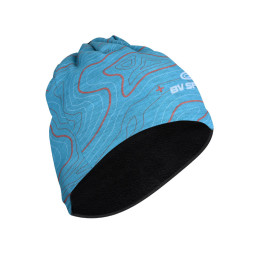• One Size• S
• M
• L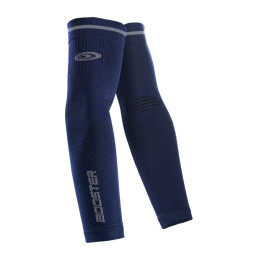• S
• M
• L• L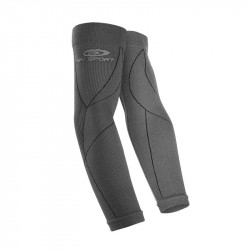• S/M
• L/XL• S/M
• L/XL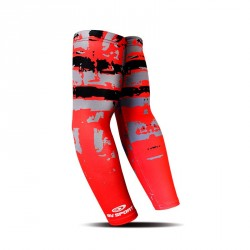• L/XL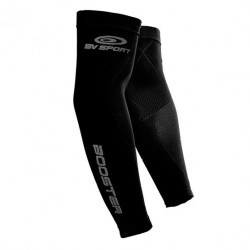• S/M
• L/XL• S/M
• L/XL• L/XL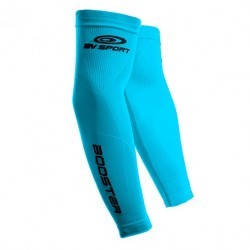• L/XL• S/M• S• S
• M
• L
• XL• S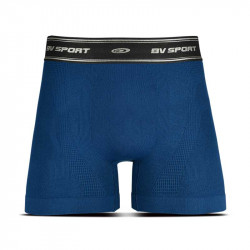• S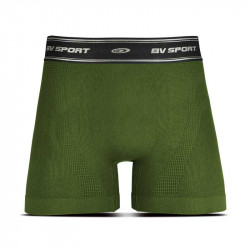• S• One Size• One Size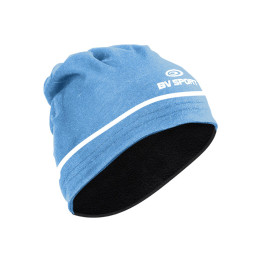• One Size• One Size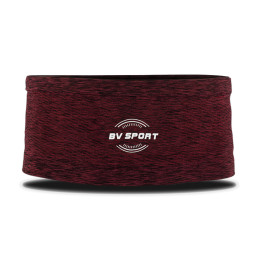• S
• M
• L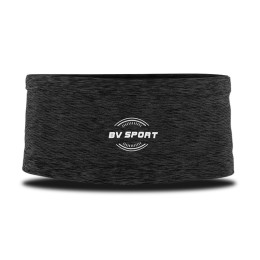• S
• M
• L